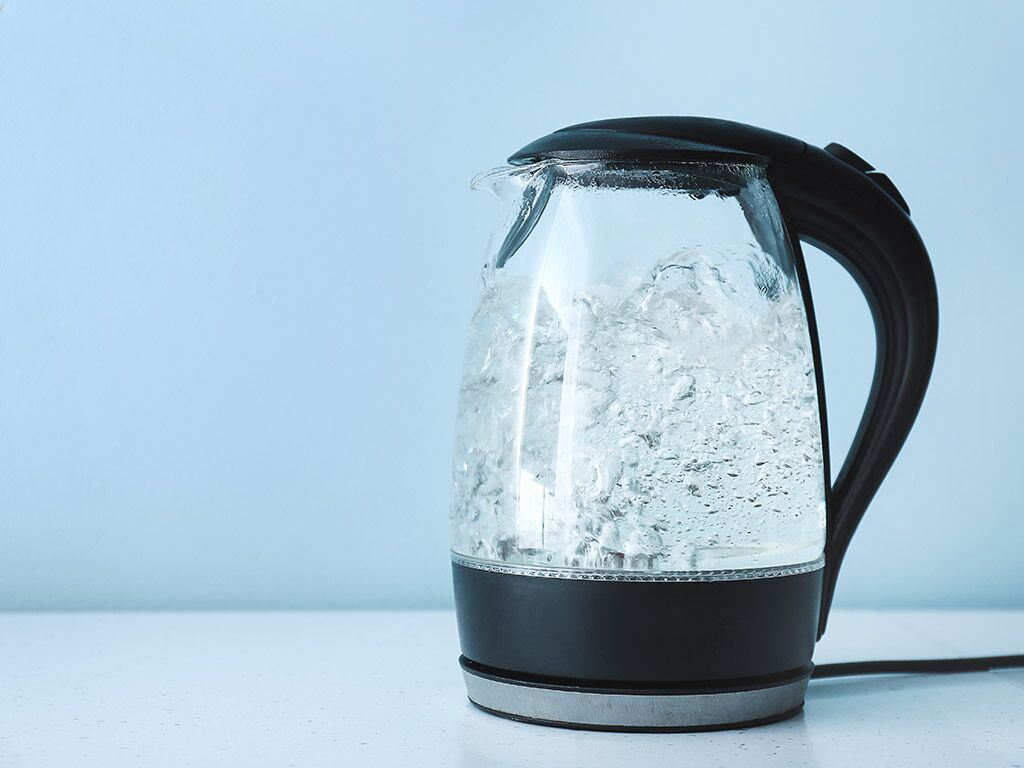# Specific Heat Study Guide

INTRODUCTION

It’s always amazing to think about how refreshing a good cool glass of water can make us feel after a long and exhausting day. We’ve all heard that water helps to regulate our body’s temperature and consequently makes us feel better. However, how do you suppose water accomplishes this? We’ll delve into the property of water having an extraordinarily high specific heat capacity to solve this topic.Source

## WHAT IS HEAT CAPACITY?

• Heat, also referred to as thermal energy, is a type of energy.
• It is possible to change the state of energy, but it can never be created or destroyed.
• The higher the temperature of a material, the more thermal energy it has, according to basic thermodynamics.
• The ratio of the amount of energy supplied to a substance to the change in temperature produced is known as heat capacity or Cp.
• Heat capacity’s formula is given as :

Heat capacity = Q/∆t,

Where Q is the quantity absorbed, and ∆t is the rise in temperature.

## WHAT IS SPECIFIC HEAT CAPACITY?

• The definition of specific heat states that it is the quantity of heat required to make one unit of mass of a substance one degree hotter.

• The relation between the heat capacity and the specific heat capacity is that the heat capacity of a material is called specific heat capacity or specific heat when the mass of the material is unity ( Heat capacity = Specific heat x mass).

• Some things heat up quickly, whereas others take a long time to heat up.

• Water is one of the latter; it has a high specific heat capacity since increasing its temperature takes more energy.

• 4182 J/kg°C is the specific heat capacity of water.Source

Specific heat capacity’s formula is

Q = C m ∆t

Where,

• Q = quantity of heat absorbed.
• m = mass of
• ∆t = Rise in temperature
• C = Specific heat capacity of a substance ( depends on the nature of the substance)

## SI UNIT OF SPECIFIC HEAT CAPACITY

J kg-1 K-1 is the unit of specific heat capacity.

## SUMMARY

• Heat capacity is the ratio of the amount of energy transferred to the change in temperature.
• The amount of heat required to raise the temperature per unit mass is known as specific heat capacity.
• The formula of specific heat capacity is Q = C m ∆t

## FAQs

Q. What is the specific heat of a substance?

Specific heat states that it is the quantity of heat required to make one unit of mass of a substance one degree hotter.

Q. How do you measure specific heat?

Specific heat can be measured using the formula Q = C m ∆t

Q. What is the difference between heat capacity and specific heat capacity?

The heat capacity of a material is called specific heat capacity or specific heat when the mass of the material is unity.

Q. Why is the specific heat capacity of water higher than metal?

The specific heat capacity of water is higher than metal because it takes more energy to raise the temperature.

We hope you enjoyed studying this lesson and learned something cool about Specific Heat! Join our Discord community to get any questions you may have answered and to engage with other students just like you! Don’t forget to download our App to experience our fun VR classrooms – we promise, it makes studying much more fun!😎

## REFERENCE

1. Specific Heat: https://www.ck12.org/physics/specific-heat/lesson/Specific-Heat-MS-PS/?referrer=concept_details. Accessed 11th April 2022.
2. Specific Heat Capacity: https://en.wikipedia.org/wiki/Specific_heat_capacity. Accessed 11th April 2022.
]]>